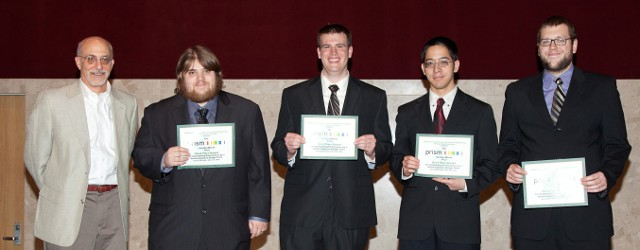# Kenji AonoNSF Graduate Fellow

## Journal Publication

Aono's Jump-Resonance based Speaker Recognition paper to appear in IEEE Transactions of Biomedical Circuits and Systems.

## Conference Publication

Aono gave a lecture at the 2012 IEEE 55th International Midwest Symposium on Circuits and Systems in Boise, ID. Paper available through IEEE Xplore.

## Graduate Fellowship

Aono has been awarded the Graduate Fellowship from the Michigan Space Grant Consortium (MSGC) for the 2012-2013 year. The Space Grant was established by Congress in 1988 and implemented by the National Aeronautics and Space Administration (NASA) in 1989. This fellowship will foster awareness of, education in, and research on space-related science and technology that reflects NASA strategic interests. Press release available here.

## Completed Undergraduate Work

The senior design project (CAPSTONE) has been successfully completed, which was awarded the Prism Prize. This concludes the undergraduate work for a B.S. in computer engineering, and a B.S. in electrical engineering.## Testing Math

Given the quadratic equation $a{x}^{2}+bx+c=0$ , the roots can be found using: $x=\frac{-b±\sqrt{{b}^{2}-4ac}}{2a}$ .

When $a \ne 0$, there are two solutions to $$ax^2 + bx + c = 0$$ and they are $$x = {-b \pm \sqrt{b^2-4ac} \over 2a}.$$

$$\begin{eqnarray} {\delta y \over \delta x} & = & {{a\over b}\over c}\\ \lim_{x\to\infty} \sum_{i=1}^{\infty}\prod_{n=1}^5 \tfrac{n^3}{i-x} & = & \sqrt{3} \dbinom{n-1}{r-1} \frac{1}{4\pi}\oint_\Sigma\frac{1}{r}\frac{\partial U}{\partial n} ds \end{eqnarray}$$

$1+\frac{1}{2}+\frac{1}{4}+\cdots = \sum_{n=1}^\infty 2^{1-n} = 2$

Firefox 3 does not handle eqnarray correctly.

This is a test for checking the handling of inline math equation: $$1+\exp(-j\omega)$$, which shouldn't mess up the spacing between lines too much.

## Greetings

This is a place-holder website, it will be updated once Aono has something to say.